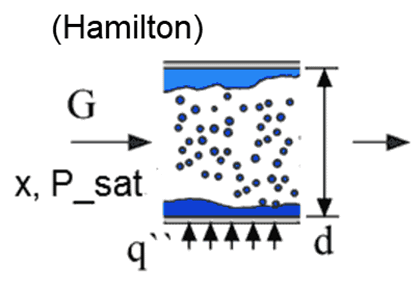Contents - Index

Flow_Boiling_HamiltonProcedure Flow_Boiling_Hamilton(Fluid\$,P_sat, G, d, x, q``: h,  T_w ) implements the Hamilton correlation to determine the heat transfer coefficient for an evaporating refrigerant in a micro-tube heat exchanger.  The correlation is applicable to pure refrigernants and blends.  The correction for blends uses the approximation reported in Kedzierski et al. (2015).

Inputs

Fluid\$ - string variable representing a real fluid in the EES data base.

P_sat - the saturation pressure of the incoming vapor in  [Pa, kPa, bar, MPa, psi,atm] (units depend on unit system selected in EES).

G - mass velocity, i.e., the ratio of the mass flow rate to the cross-sectional area of the tube [kg/s-m^2] or [lbm/hr-ft^2]

d - diameter of the tube [m] or [ft].

x - quality (must be between 0 and 1)

q`` - surface heat flux [W/m^2] or [Btu/hr-ft^2]

Outputs

h - heat transfer coefficient [W/m^2-K] or [Btu/hr-ft^2-F] including convective and nucleate boiling contributions.

T_w - estimated temperature of the inside surface of the tube in [C], [K], [F], or [R].

Notes:

1.  This procedure is responsible for determining the property data of the specified fluid . Fluid\$ must be a provided with a string variable or string constant that is one of the (non-ideal gas) fluids in EES.  It uses the correlation developed by Shah (1982).

2.   At x=1, the procedure returns that heat transfer coefficient expected for a single phase vapor at the given mass velocity and diameter

3.   At high values of quality that would result in a Reynold's number less than 2300, linear interpolation is used between the heat transfer coefficient at a quality that results in Re=2300 and the x=1 value.

4.   This function was originally named Flow_Boiling but has been renamed to Flow_Boiling_Shah to distinguish it form the Flow_Boiling_Chen correlation.  Calling Flow_Boiling will still work for backward compatibility.

Example:

\$UnitSystem SI K Pa J

F\$='R22'

T_sat=250 [K]                                "boiling saturation temperature"

P_sat=pressure(F\$,T=T_sat,x=x)    "saturation pressure"

G=200 [kg/m^2-s]                           "mass velocity"

d=0.0172 [m]                                  "tube inner diameter"

x=0.05                                           "quality"

\$VarInfo q`` lower=100 guess=10000

Call flow_boiling_hamilton(F\$, P_sat, G,  d, x, q``: h,  T_w)

q``=U*(T_h-T_sat)                          "heat flux"

T_h=261 [K]                                   "temperature of fluid on outside of pipe"

h_h=5400 [W/m^2-K]                      "heat transfer coefficient on outside surface of pipe"

U=(1/h+1/h_h)^(-1)                         "overall heat transfer coefficient between fluids on either side of the pipe wall"

{Solution

h=1357 [W/m^2-K]

P_sat=216937 [Pa]

q``=11929 [W/m^2]

T_w=258.8 [K]

U=1084 [W/m^2-K]}